# SAT Math Multiple Choice Question 897: Answer and Explanation

### Test Information

Question: 897

12.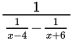Which of the following is equivalent to the expression above?

• A. 10
• B.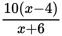• C.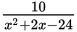• D.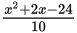Explanation:

D

Difficulty: Medium

Category: Passport to Advanced Math / Exponents

Strategic Advice: Questions that involve "complex" fractions (fractions within fractions) require patience and strategy. Ignore the big "1 over" part initially. If you rewrite the messy denominator as a single fraction, then you can simply "flip it" to get the final answer.

Getting to the Answer: To combine the two terms in the denominator, find the least common denominator, (x - 4)(x + 6), and write each term as a fraction with that denominator. Then, simplify as needed by using FOIL and combining like terms: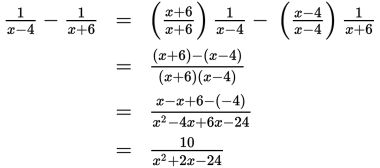Don't answer too quickly! You still need to take the reciprocal of the simplified expression to perform the final "1 over" part of the original expression, making (D) the correct answer.